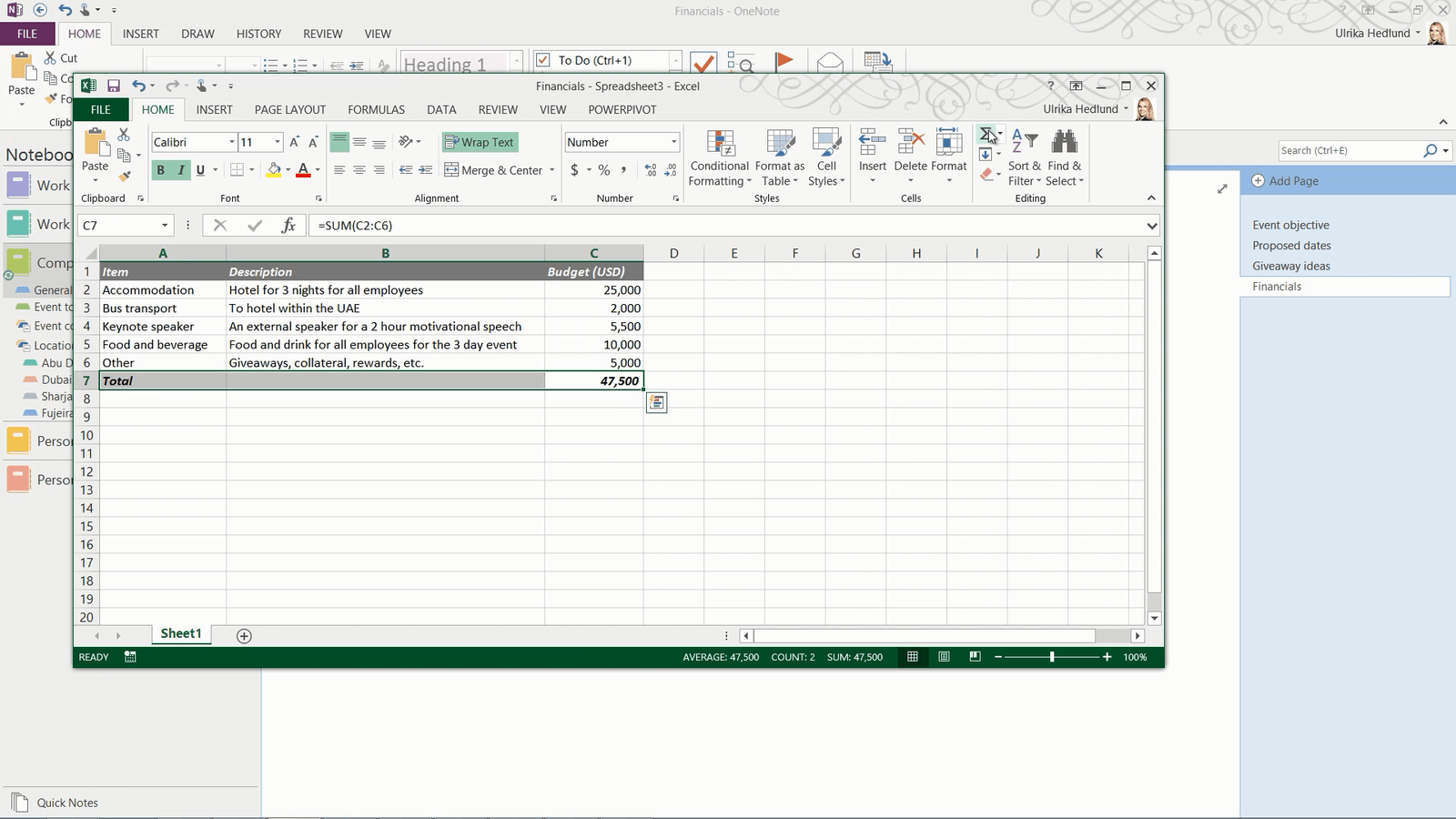# How to perform calculations

Posted on

To calculate numbers, just type what you want to calculate using common math operators. Here for instance I want to know what the total accommodation budget is broken down per day, so I’ll type in 25000 divided by 3 equals, and OneNote shows the result.This works fine for simple calculations, but quite often you need to perform more advanced calculations. Here for instance I would like to calculate the total budget.

In OneNote 2013 you now have support for Excel spreadsheets. To convert a table to a spreadsheet, place your marker anywhere in your table. On the “TABLE TOOLS” “LAYOUT” tab, click “Convert to Excel Spreadsheet”.To edit the spreadsheet click “Edit” in the top left corner. Now you can use all the familiar calculation tools in Excel.Then I’ll save my changes and close down the spreadsheet and the changes are updated in my notes.